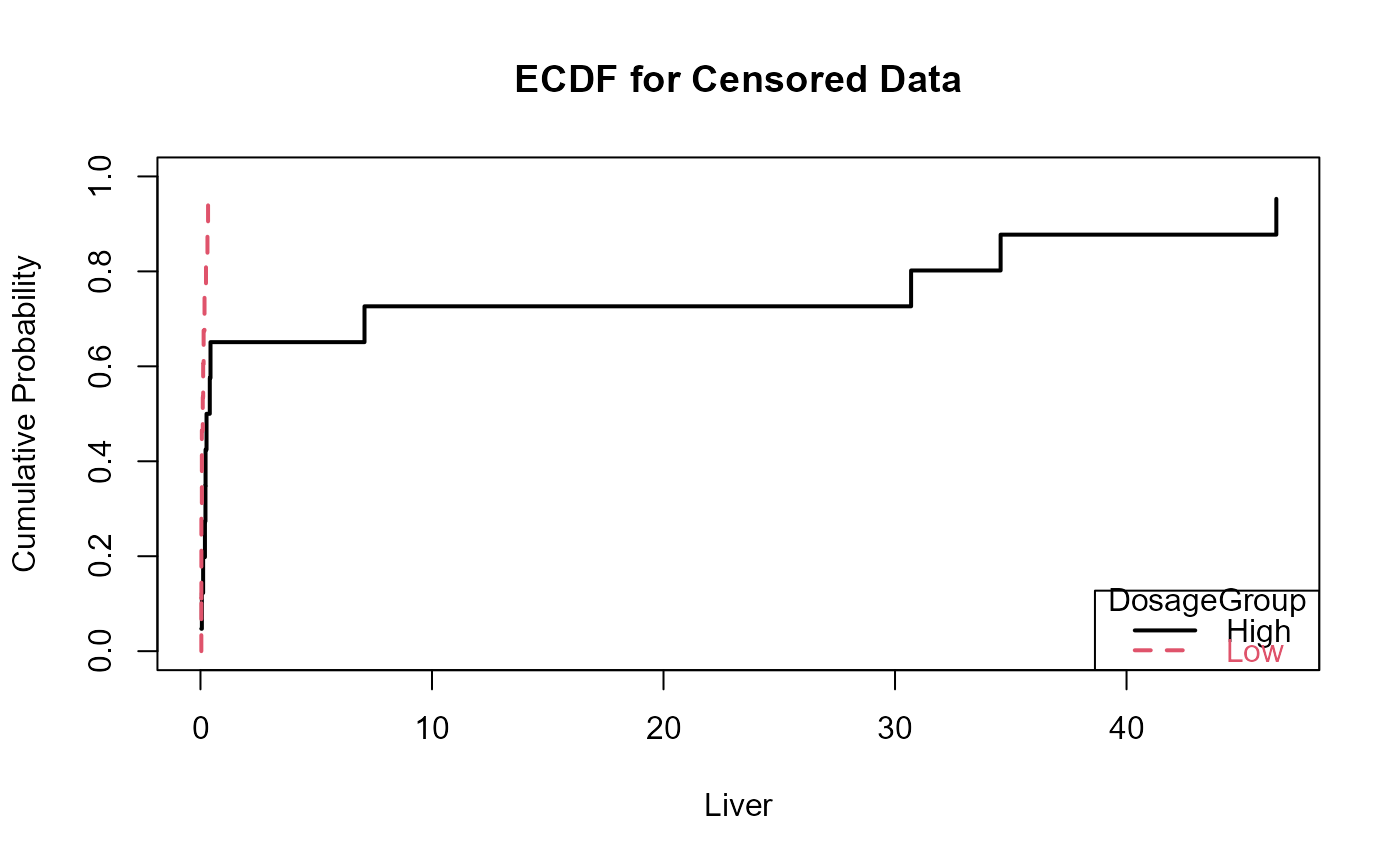Plots ecdfs of one or more groups of censored data. Illustrates the differences between groups for group tests such as those done using cen1way or cenanova.

cen_ecdf(
x.var,
cens.var,
xgroup = NULL,
xlim = c(0, max(y.var)),
Ylab = varname
)

## Arguments

x.var

The column of data values plus detection limits

cens.var

The column of indicators, where 1 (or TRUE) indicates a detection limit in the y.var column, and 0 (or FALSE) indicates a detected value in y.var.

xgroup

Optional - grouping or factor variable. Can be either a text or numeric value indicating the group assignment.

xlim

Limits for the x (data) axis of the ecdf plot. Default is 0 to the maximum of the y.var variable. To change, use option xlim = c(0, 50) if 50 is to be the maximum on the plot.

Ylab

Optional – input text in quotes to be used as the variable name on the ecdf plot. The default is the name of the y.var input variable.

## Value

Plots an empirical cumulative distribution function. If group=NULL the ECDF of the entire dataset is produced. If group is identified then ECDFs are plotted for each group seperately and a legend added.

## Examples

data(PbHeron)

# with groups
with(PbHeron, cen_ecdf(Liver, LiverCen, DosageGroup))# all data
with(PbHeron, cen_ecdf(Liver, LiverCen))